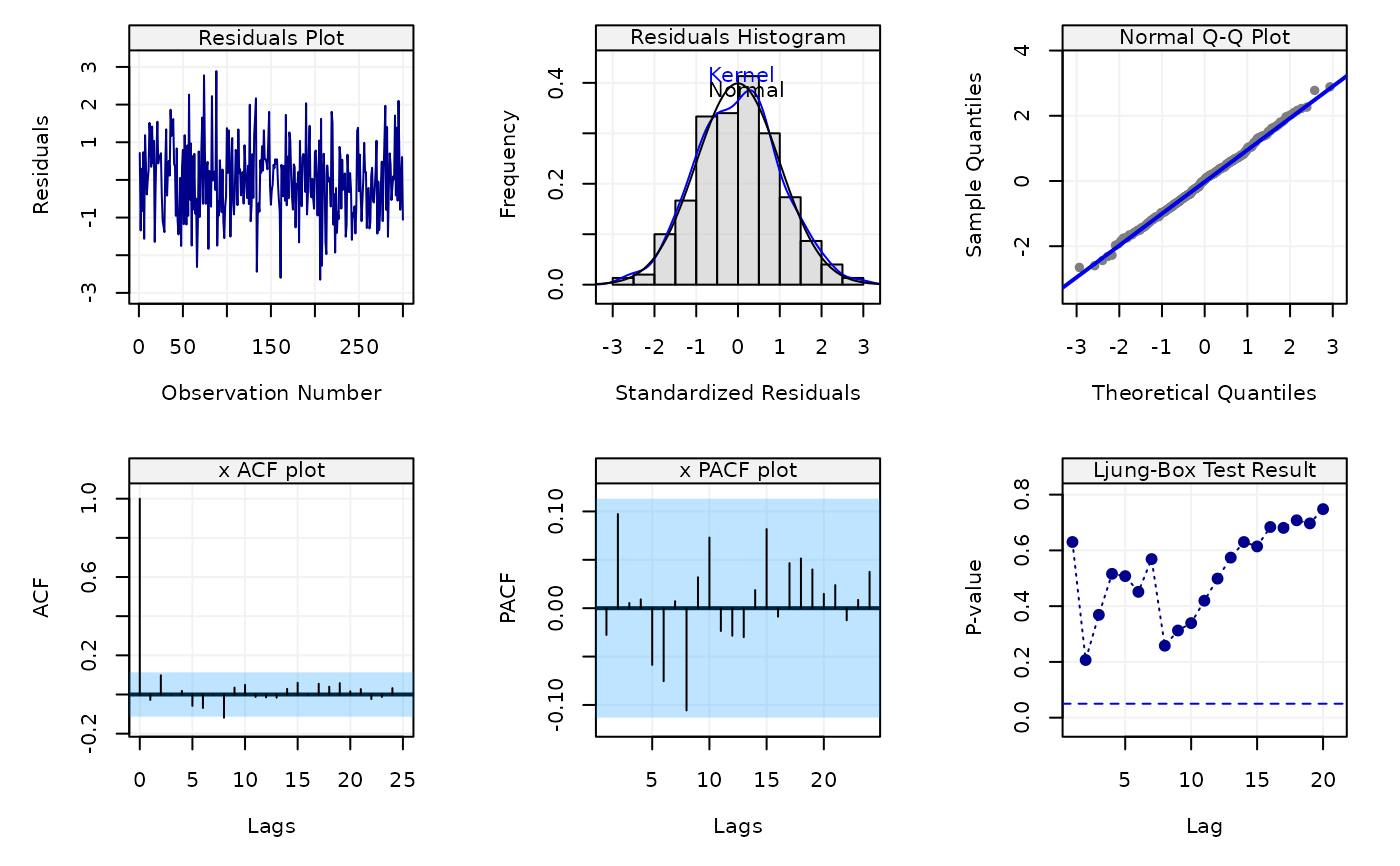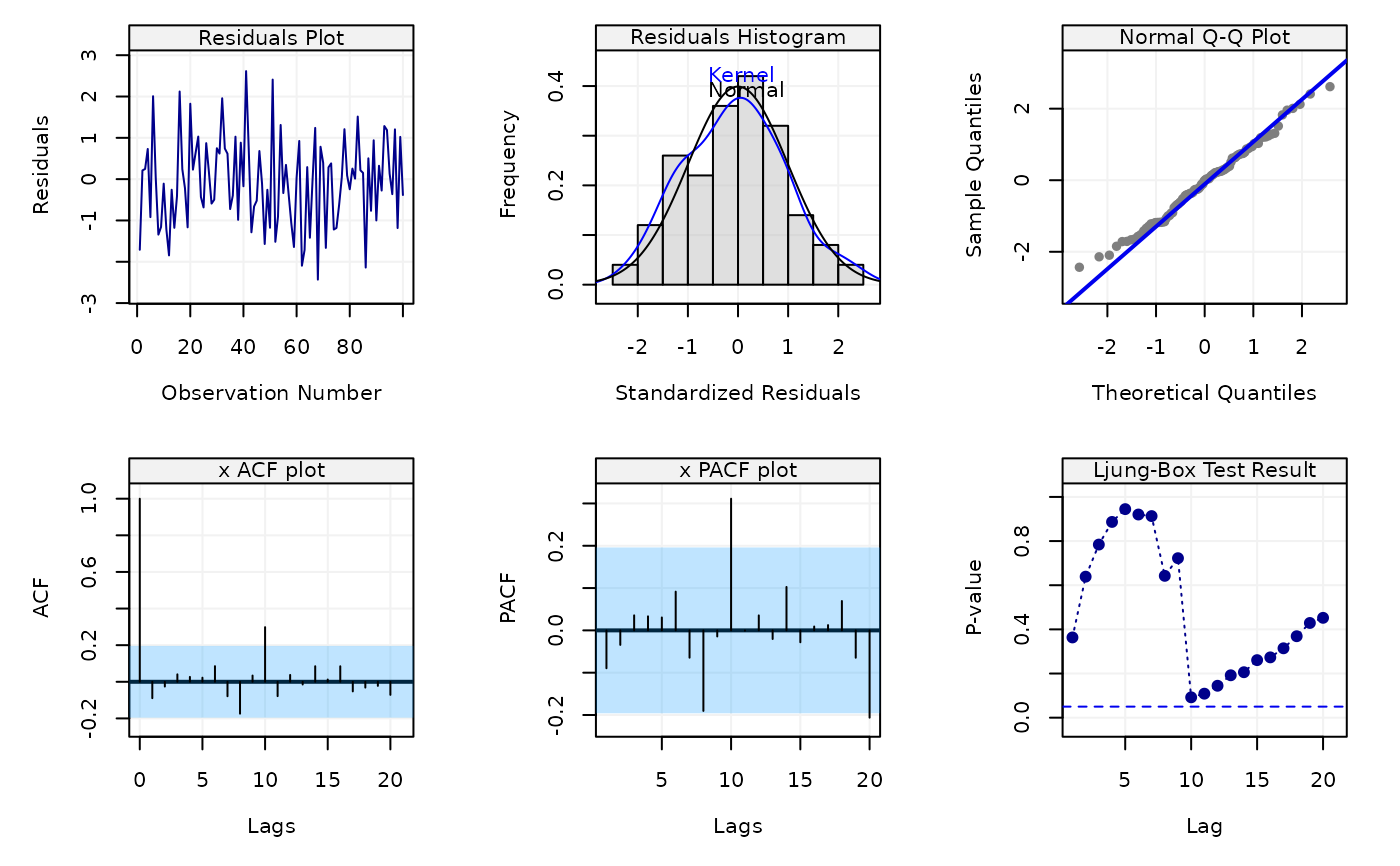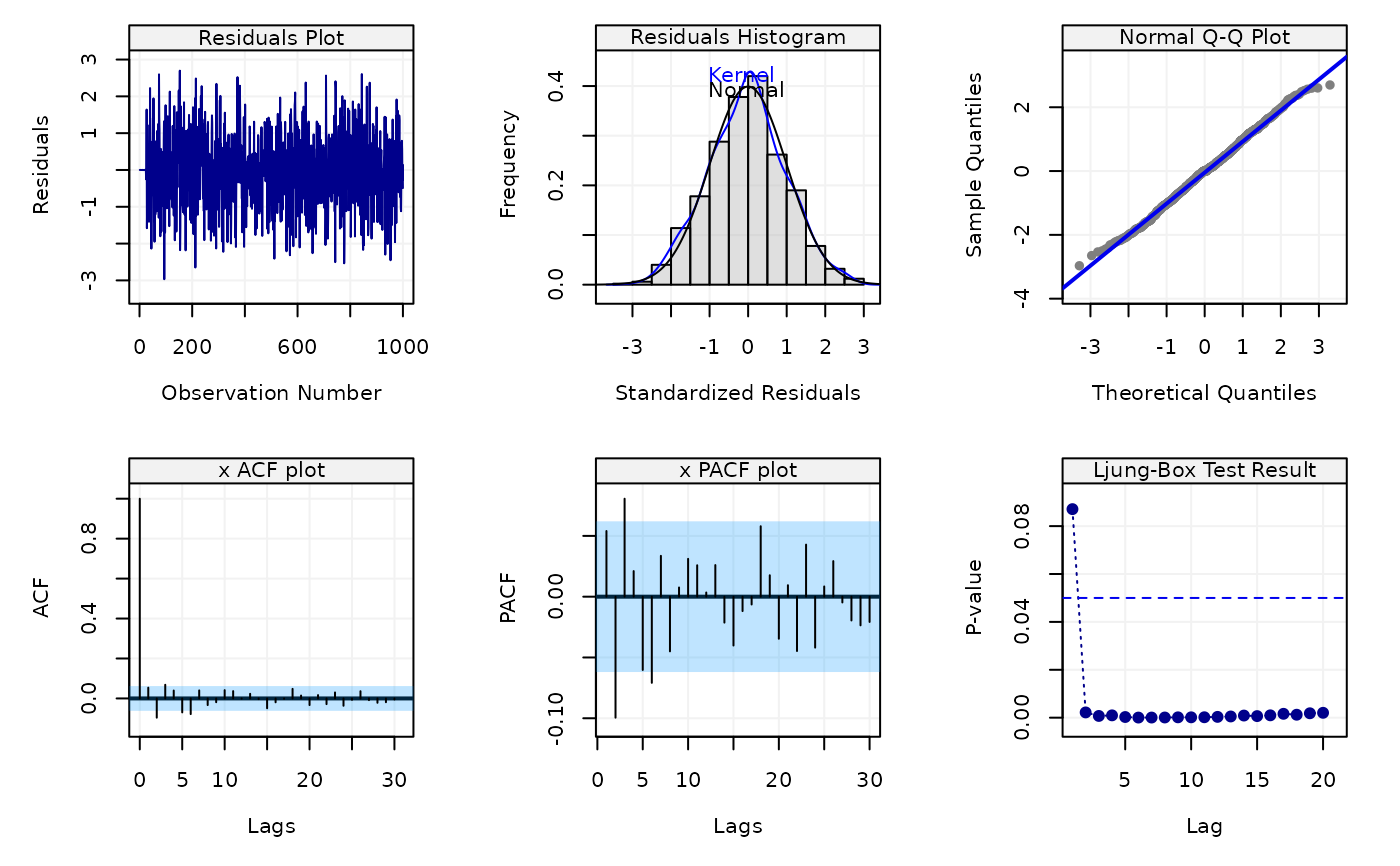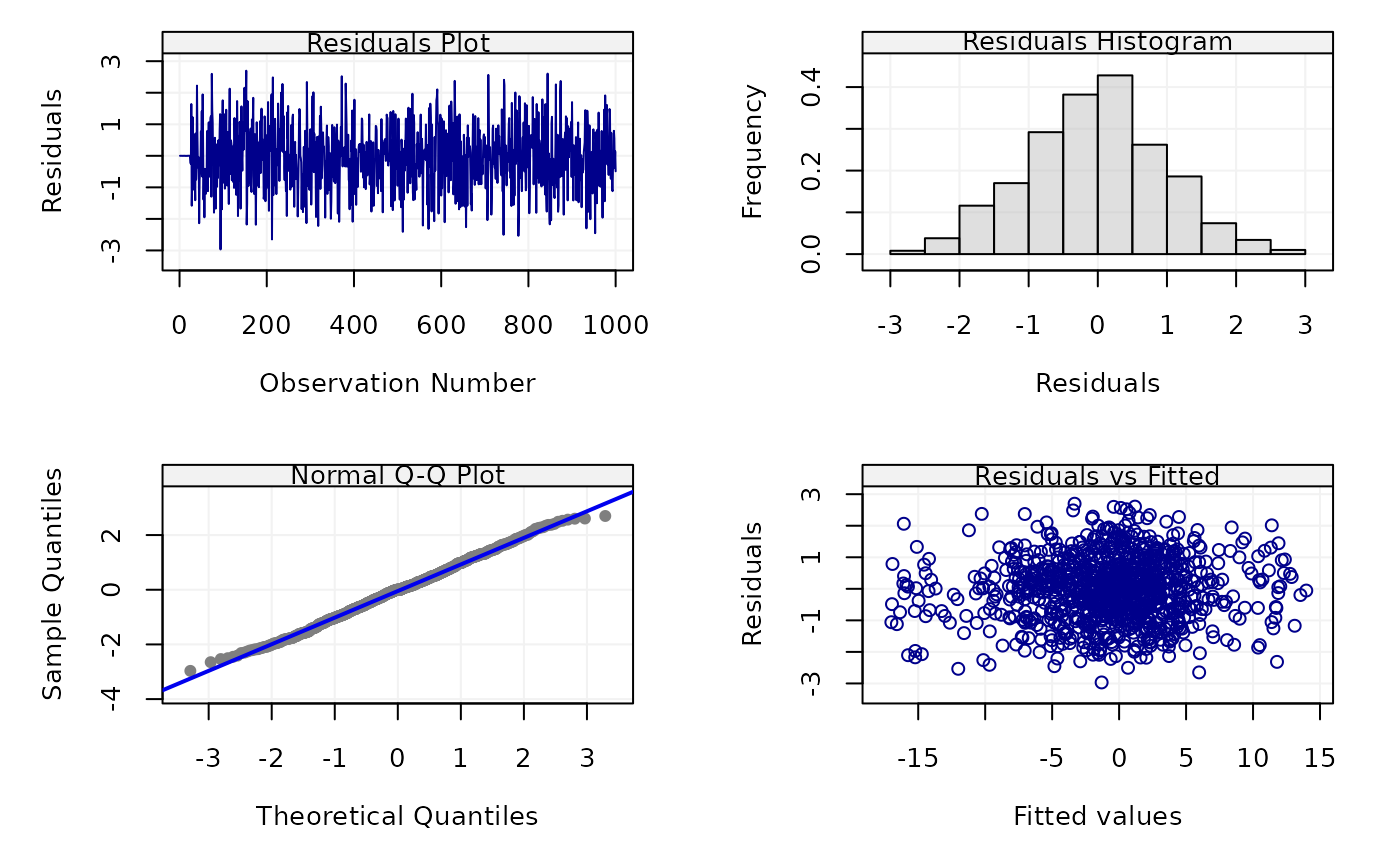This function can perform (simple) diagnostics on the fitted time series model. It can output 6 diagnostic plots to assess the model, including (1) residuals plot, (2) histogram of distribution of standardized residuals, (3) Normal Q-Q plot of residuals, (4) ACF plot, (5) PACF plot, (6) Box test results.

check(model = NULL, resids = NULL, simple = FALSE)

## Arguments

model

A fitsimts, lm or gam object.

resids

A vector of residuals for diagnostics.

simple

A boolean indicating whether to return simple diagnostic plots or not.

## Author

Stéphane Guerrier and Yuming Zhang

## Examples

Xt = gen_gts(300, AR(phi = c(0, 0, 0.8), sigma2 = 1))
model = estimate(AR(3), Xt)
check(model)check(resids = rnorm(100))Xt = gen_gts(1000, SARIMA(ar = c(0.5, -0.25), i = 0, ma = 0.5, sar = -0.8,
si = 1, sma = 0.25, s = 24, sigma2 = 1))
model = estimate(SARIMA(ar = 2, i = 0, ma = 1, sar = 1, si = 1, sma = 1, s = 24),
Xt, method = "rgmwm")
check(model)check(model, simple=TRUE)